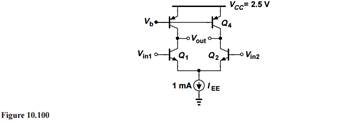### Create an Account

Home / Questions / Consider the differential amplifier shown in Fig 10100 where the input CM level is equal t...

# Consider the differential amplifier shown in Fig 10100 where the input CM level is equal to 1.2 V Adjust the value of Vb so as to set the output CM level to 1 5 Determine the small-

Consider the differential amplifier shown in Fig. 10.100, where the input CM level is equal to 1.2 V.(a) Adjust the value of Vb so as to set the output CM level to 1.5 V.

(b) Determine the small-signal differential gain of the circuit. (Hint: to provide differential inputs, use an independent voltage source for one side and a voltage-dependent voltage source for the other.)

(c) What happens to the output CM level and the gain if Vb varies by ±10 mV?

Apr 24 2020 View more View LessSubscribe To Get Solution